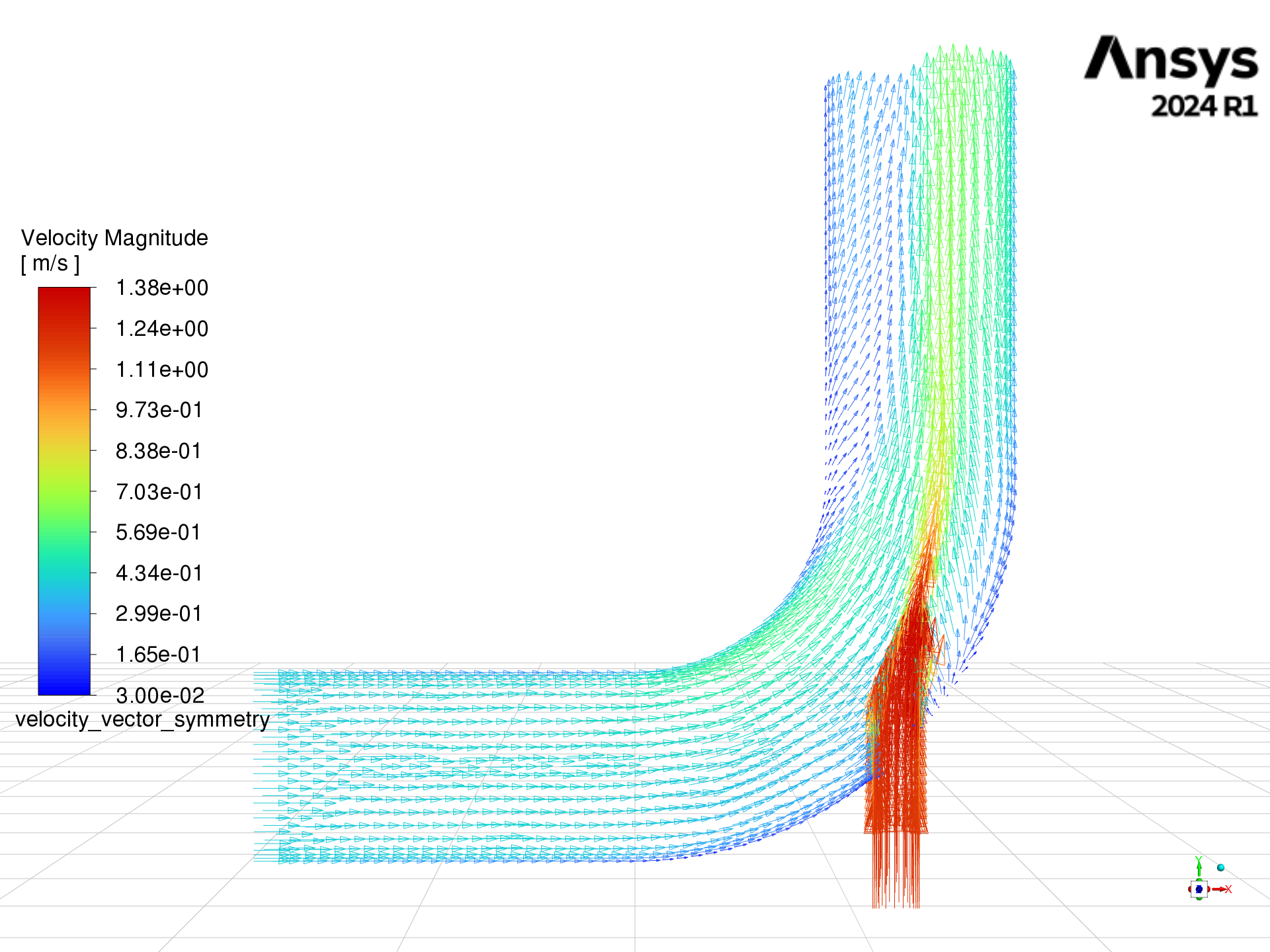# Fluent setup and solution using settings objects#

This example sets up and solves a three-dimensional turbulent fluid flow and heat transfer problem in a mixing elbow, which is common in piping systems in power plants and process industries. Predicting the flow field and temperature field in the area of the mixing region is important to designing the junction properly.

This example uses settings objects.

Problem description

A cold fluid at 20 deg C flows into the pipe through a large inlet. It then mixes with a warmer fluid at 40 deg C that enters through a smaller inlet located at the elbow. The pipe dimensions are in inches, and the fluid properties and boundary conditions are given in SI units. Because the Reynolds number for the flow at the larger inlet is `50, 800`, a turbulent flow model is required.

```# sphinx_gallery_thumbnail_path = '_static/mixing_elbow_settings.png'
```

## Perform required imports#

```import ansys.fluent.core as pyfluent
from ansys.fluent.core import examples

```

## Launch Fluent#

Launch Fluent as a service in meshing mode with double precision running on two processors.

```solver = pyfluent.launch_fluent(precision="double", processor_count=2, mode="solver")
```

## Import mesh and perform mesh check#

Import the mesh and perform a mesh check, which lists the minimum and maximum x, y, and z values from the mesh in the default SI units of meters. The mesh check also reports a number of other mesh features that are checked. Any errors in the mesh are reported. Ensure that the minimum volume is not negative because Fluent cannot begin a calculation when this is the case.

```solver.file.read(file_type="case", file_name=import_filename)
solver.tui.mesh.check()
```

## Set working units for mesh#

Set the working units for the mesh to inches. Because the default SI units are used for everything except length, you do not have to change any other units in this example. If you want working units for length to be other than inches (for example, millimeters), make the appropriate change.

```solver.tui.define.units("length", "in")
```

## Enable heat transfer#

Enable heat transfer by activating the energy equation.

```solver.setup.models.energy.enabled = True
```

## Create material#

Create a material named `"water-liquid"`.

```if solver.get_fluent_version() == "22.2.0":
solver.setup.materials.copy_database_material_by_name(
type="fluid", name="water-liquid"
)
else:
solver.setup.materials.database.copy_by_name(type="fluid", name="water-liquid")
```

## Set up cell zone conditions#

Set up the cell zone conditions for the fluid zone (`elbow-fluid`). Set `material` to `"water-liquid"`.

```solver.setup.cell_zone_conditions.fluid["elbow-fluid"].material = "water-liquid"
```

## Set up boundary conditions for CFD analysis#

Set up the boundary conditions for the inlets, outlet, and walls for CFD analysis.

```# cold inlet (cold-inlet), Setting: Value:
# Velocity Specification Method: Magnitude, Normal to Boundary
# Velocity Magnitude: 0.4 [m/s]
# Specification Method: Intensity and Hydraulic Diameter
# Turbulent Intensity: 5 [%]
# Hydraulic Diameter: 4 [inch]
# Temperature: 293.15 [K]

if solver.get_fluent_version() == "22.2.0":
solver.setup.boundary_conditions.velocity_inlet["cold-inlet"].vmag = {
"option": "constant or expression",
"constant": 0.4,
}
else:
solver.setup.boundary_conditions.velocity_inlet["cold-inlet"].vmag = 0.4
solver.setup.boundary_conditions.velocity_inlet[
"cold-inlet"
].ke_spec = "Intensity and Hydraulic Diameter"
solver.setup.boundary_conditions.velocity_inlet["cold-inlet"].turb_intensity = 0.05
solver.setup.boundary_conditions.velocity_inlet[
"cold-inlet"
].turb_hydraulic_diam = "4 [in]"
if solver.get_fluent_version() == "22.2.0":
solver.setup.boundary_conditions.velocity_inlet["cold-inlet"].t = {
"option": "constant or expression",
"constant": 293.15,
}
else:
solver.setup.boundary_conditions.velocity_inlet["cold-inlet"].t = 293.15

# hot inlet (hot-inlet), Setting: Value:
# Velocity Specification Method: Magnitude, Normal to Boundary
# Velocity Magnitude: 1.2 [m/s]
# Specification Method: Intensity and Hydraulic Diameter
# Turbulent Intensity: 5 [%]
# Hydraulic Diameter: 1 [inch]
# Temperature: 313.15 [K]

if solver.get_fluent_version() == "22.2.0":
solver.setup.boundary_conditions.velocity_inlet["hot-inlet"].vmag = {
"option": "constant or expression",
"constant": 1.2,
}
else:
solver.setup.boundary_conditions.velocity_inlet["hot-inlet"].vmag = 1.2
solver.setup.boundary_conditions.velocity_inlet[
"hot-inlet"
].ke_spec = "Intensity and Hydraulic Diameter"
solver.setup.boundary_conditions.velocity_inlet[
"hot-inlet"
].turb_hydraulic_diam = "1 [in]"
if solver.get_fluent_version() == "22.2.0":
solver.setup.boundary_conditions.velocity_inlet["hot-inlet"].t = {
"option": "constant or expression",
"constant": 313.15,
}
else:
solver.setup.boundary_conditions.velocity_inlet["hot-inlet"].t = 313.15

# pressure outlet (outlet), Setting: Value:
# Backflow Turbulent Intensity: 5 [%]
# Backflow Turbulent Viscosity Ratio: 4

solver.setup.boundary_conditions.pressure_outlet["outlet"].turb_viscosity_ratio = 4
```

## Disable plotting of residuals during calculation#

Disable plotting of residuals during the calculation.

```solver.tui.solve.monitors.residual.plot("no")
```

## Initialize flow field#

Initialize the flow field using hybrid initialization.

```solver.solution.initialization.hybrid_initialize()
```

## Solve for 150 iterations#

Solve for 150 iterations.

```solver.solution.run_calculation.iterate.get_attr("arguments")
if solver.get_fluent_version() >= "23.1.0":
solver.solution.run_calculation.iterate(iter_count=150)
else:
solver.solution.run_calculation.iterate(number_of_iterations=150)
```

## Create velocity vectors#

Create and display velocity vectors on the `symmetry-xyplane` plane.

```solver.results.graphics.vector["velocity_vector_symmetry"] = {}
solver.results.graphics.vector["velocity_vector_symmetry"].print_state()
solver.results.graphics.vector["velocity_vector_symmetry"].field = "temperature"
solver.results.graphics.vector["velocity_vector_symmetry"].surfaces_list = [
"symmetry-xyplane",
]
solver.results.graphics.vector["velocity_vector_symmetry"].scale.scale_f = 4
solver.results.graphics.vector["velocity_vector_symmetry"].style = "arrow"
```
```name : velocity_vector_symmetry
field : velocity-magnitude
vector_field : velocity
scale :
auto_scale : True
scale_f : 1
style : 3d arrow
skip : 0
vector_opt :
in_plane : False
fixed_length : False
x_comp : True
y_comp : True
z_comp : True
color :
range_option :
option : auto-range-on
auto_range_on :
global_range : True
color_map :
visible : True
size : 100
color : field-velocity
log_scale : False
format : %0.2e
user_skip : 9
show_all : True
position : 1
font_name : Helvetica
font_automatic : True
font_size : 0.032
length : 0.54
width : 6.0
display_state_name : None
```## Compute mass flow rate#

Compute the mass flow rate.

```solver.solution.report_definitions.flux["mass_flow_rate"] = {}
solver.solution.report_definitions.flux["mass_flow_rate"].zone_names.get_attr(
"allowed-values"
)
solver.solution.report_definitions.flux["mass_flow_rate"].zone_names = [
"cold-inlet",
"hot-inlet",
"outlet",
]
solver.solution.report_definitions.flux["mass_flow_rate"].print_state()
solver.solution.report_definitions.compute(report_defs=["mass_flow_rate"])
```
```report_type : flux-massflow
average_over : 1
per_zone : False
zone_names :
0 : cold-inlet
1 : hot-inlet
2 : outlet

[{'mass_flow_rate': [-9.568956841343734e-06, 0]}]
```

## Close Fluent#

Close Fluent.

```solver.exit()
```

Total running time of the script: ( 0 minutes 45.661 seconds)

Gallery generated by Sphinx-Gallery